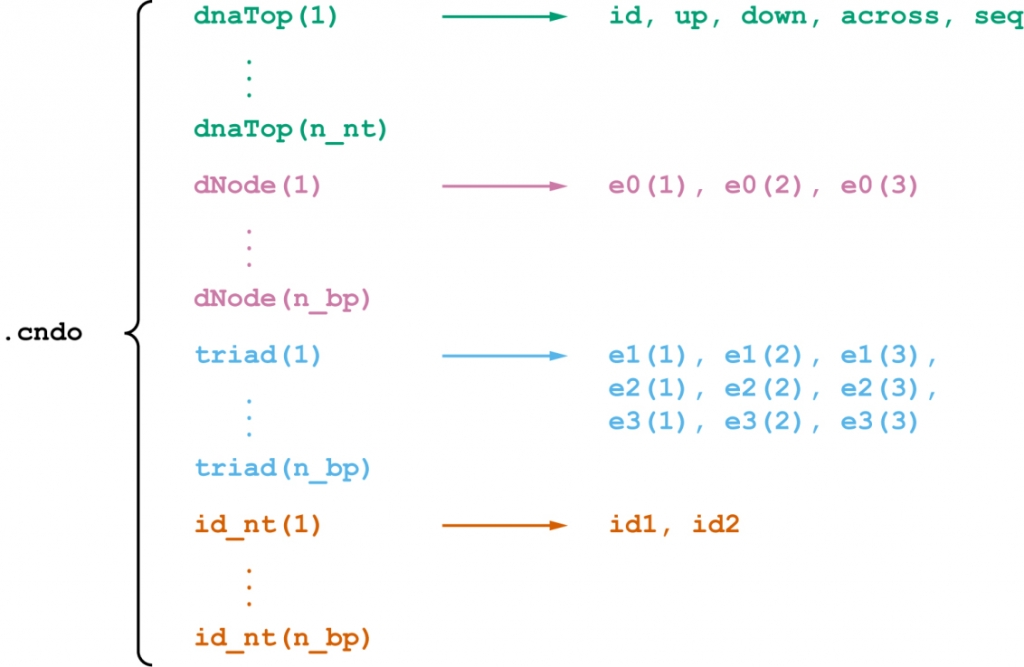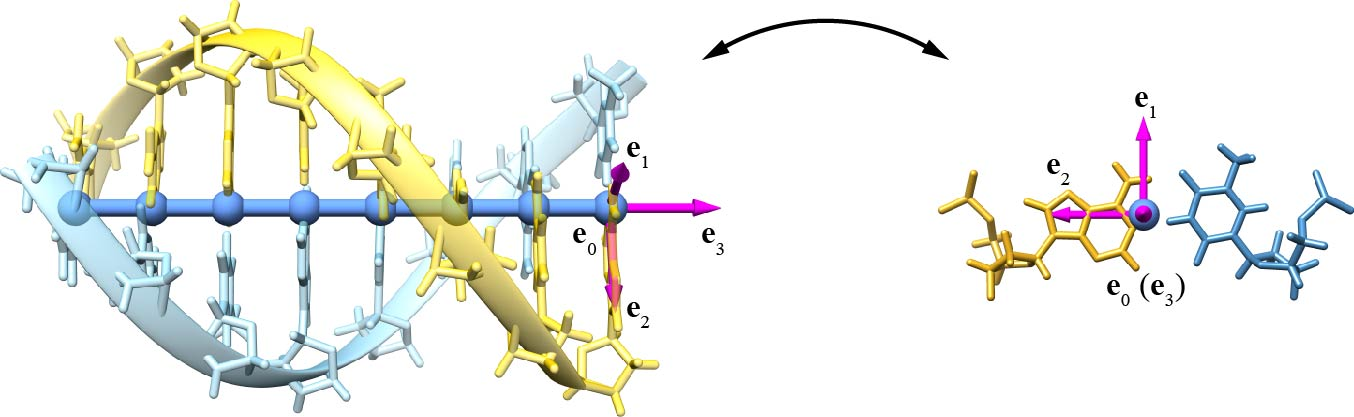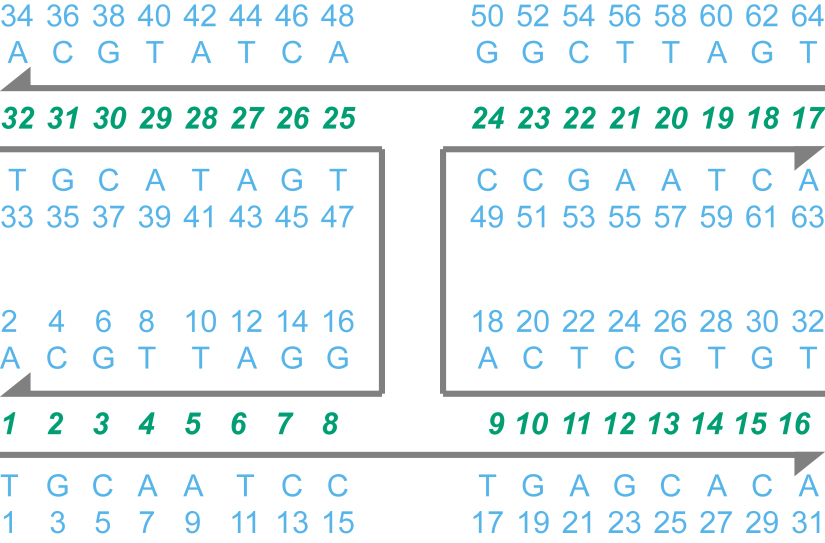We have developed the CanDo file format (.cndo) that describes the sequence, topology, and geometry of a programmed DNA assembly. We also provide a set of MATLAB scripts that convert a caDNAno design to a .cndo file.

Users of this tool are kindly requested to cite the following reference:

• K Pan, DN Kim, F Zhang, MR Adendorff, H Yan, M Bathe. Lattice-free prediction of three-dimensional structure of programmed DNA assemblies. Nature Communications, 5: 5578 (2014). [ PubMed Article ]

#### Example: A DX tile

Here we use a double crossover (DX) tile designed using the software caDNAno  on a honeycomb  lattice to demonstrate the use of this .cndo file converter. The secondary structure was stored in the file tutorial_v2.json, and the nucleotide sequences were in the file tutorial_v2.csv. The following steps are run in MATLAB.

Step 2. Run the MATLAB function json2cndo.m. The syntax of this function is

[] = json2cndo(jsonPATH, csvPATH, cndoPATH, latticeType)

The first and second arguments are the paths to the .json and .csv files, respectively. The third argument is the path to the .cndo file to be created. The value of the last argument should be either ‘honeycomb’ if the design is on a honeycomb  lattice or ‘square’ if the design is on a square  lattice. We assume that the .json and .csv files are saved in the current directory. Run the following command,

>> json2cndo(‘tutorial_v2.json’, ‘tutorial_v2.csv’, ‘tutorial_v2.cndo’, ‘honeycomb’);

and the new .cndo file tutorial_v2.cndo will be saved to the current directory.

Note. Sometimes the caDNAno design does not contain nucleotide sequences, and thus the .csv file is not available. In this case, leave the path to the .csv file as an empty string, such as

>> json2cndo(‘tutorial_v2.json’, ‘’, ‘tutorial_v2_noseq.cndo’, ‘honeycomb’);

and the identities of all the nucleotide will be ‘N’ in the generated file tutorial_v2_noseq.cndo.

## .cndo File Format

Let n_nt and n_bp denote the numbers of nucleotides and basepairs, respectively, in the assembly. As shown in Figure 1, the file format consists of n_nt fields dnaTop(1), …, dnaTop(n_nt) that uniquely define the sequence and topology and 3*n_bp fields dNode(1), …, dNode(n_bp), triad(1), …, triad(n_bp), id_nt(1), …, id_nt(n_bp) that uniquely defines the geometry.Figure 1. Diagram of the logical structure of the .cndo file format.

Each of the fields dnaTop(1), …, dnaTop(n_nt) represents a nucleotide and comprises five subfields. The subfield id is a positive integer serving as the unique identifier of the current nucleotide. The subfields up, down, and across are three integers as the unique identifiers of the nucleotides in the 5′-direction, that in the 3′-direction, and the complementary nucleotide, respectively. If any of these nucleotides does not exist, then the corresponding field equals –1. The subfield seq is the identity of the current nucleotide, which is ‘A’, ‘T’, ‘G’, ‘C’, or other letters.

The remaining fields represent the geometry of the DNA assembly. The field dNode(i) consists of the Cartesian coordinates e0 of the center of the reference frame for the i-th basepair. The field triad(i) stores the three axes e1, e2, and e3 of the reference frame for the i-th basepair. The reference frame is defined using the 3DNA convention (Figure 2). The field id_nt(i) consists of two elements, of which the element id1 is the unique identifier of the preferred nucleotide in the i-th basepair (yellow in Figure 2), and the element id2 is the unique identifier of the other nucleotide in the i-th basepair (blue in Figure 2).Figure 2. Definition of the center and three axes of the reference frame using the 3DNA convention. The axes e1, e2, and e3 point to the major groove, the preferred nucleotide (yellow), and along the duplex axis towards the 3′-direction of the strand with the preferred nucleotide, respectively.

A .cndo file consists of n_nt + 3*n_bp + 9 lines defined as follows:

• Line 1: A string describing the .cndo file format: “CanDo (.cndo) file format version 1.0, Keyao Pan, Laboratory for Computational Biology and Biophysics, Massachusetts Institute of Technology, November 2015”
• Line 2: an empty line
• Line 3: a string dnaTop,id,up,down,across,seq as the header of the fields dnaTop(1), …, dnaTop(n_nt)
• Line 4 – n_nt+3: six subfields separated by commas, which are the serial number (1, 2, …, n_nt), id, up, down, across, and seq.
• Line n_nt+4: an empty line
• Line n_nt+5: a string dNode,”e0(1)”,”e0(2)”,”e0(3)” as the header of the fields dNode(1), …, dNode(n_bp)
• Line n_nt+6n_nt+n_bp+5: four subfields separated by commas, which are the serial number (1, 2, …, n_bp) of the basepair and the Cartesian coordinates e0 of the center of the reference frame.
• Line n_nt+n_bp+6: an empty line